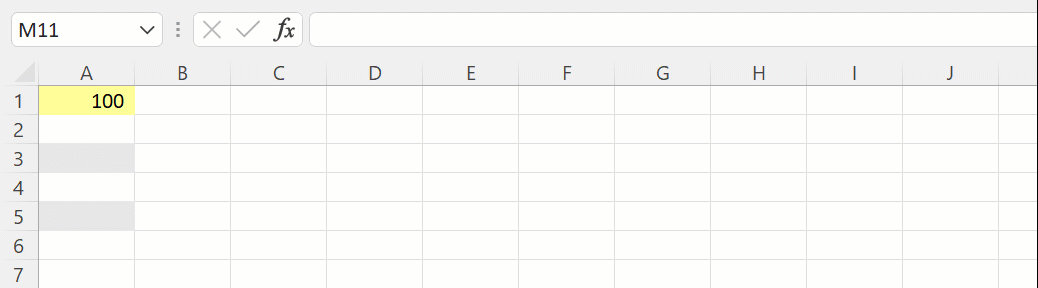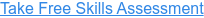Back to Blog# How to use Excel's IFS Function

Published
Sep 22, 2022 5:01:55 PM

Returns one value for a TRUE result, and another for a FALSE result for multiple conditions

#### What does Excel's IFS function do?

The IFS function is one of Excel's logical functions.

The IFS function performs a series of logical tests (that is to say - is something true or false) and then returns the value you have provided if the result is TRUE or the value you have provided if the result is FALSE.

The IFS function is an upgrade of the IF function, one of the most popular functions in Excel, as it allows financial modellers to test multiple arguments without needing a complicated nested IF formula.

In financial modelling, it is often used to model business logic based on 1, 0 flags.

#### Here's a simple example

Let's take a look at a simple example of the IFS function.

We have the following business logic:

• If the value is >=100 then return high
• If the value is >=50 then return medium
• If the value is >=10 then return low
• If the value is <10 then return extra low

Using a nested IF statement this would be structured as follows:

=IF(A1>=100,"high",IF(A1>=50,"medium",IF(A1>=10,"low","extra low")))

Using the IFS function instead of the IF function this becomes:

=IFS(A1>=100,"high",A1>=50,"medium",A1>=10,"low",A1<10,"extra low")#### What does that mean in plain English?

The best way to understand the IFS function is to replace the first comma in the pair with the word “then” and the second comma in the pair with the word “Otherwise”:

If the value in cell A1 is greater than or equal to 100, then return “High”, otherwise if it is greater than or equal to 50, then return “medium”, otherwise if it is greater than or equal to 10, then return “low” otherwise if it is less than 10, then return “ extra low”.

#### =IFS(logical test1, value if true1, logical test2, value if true2, logical test3, value if true3….)

The logical tests are in pairs: The test and the value if the result is "true":

Logical test1 – A question resulting in the answer TRUE or FALSE

Value if true1 – What to return if the answer to the logical test is TRUE

Logical test2 – A question resulting in the answer TRUE or FALSE

Value if true2 – What to return if the answer to the logical test is TRUE

#### What to consider when using the IFS function in your financial model

• The IFS function allows you to test up to 127 different conditions, but I highly recommend against going much above 4 or 5 as the tests need to be entered in the correct order and can become very difficult to review.
• The IFS function was introduced in 2016, so be aware of compatibility issues for users of previous Excel versions.
• XLOOKUP, VLOOKUP or INDEX MATCH can often provide a clearer and simpler solution to challenges often solved by multiple IF arguments.

Read more about the IFS function on the Microsoft support page here.

#### Take our free 30-question skills assessment

See where you stand with your Excel, financial modelling, data visualisation & analytics, and modelling tech skills